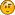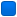###Author Topic: triangle program  (Read 2785 times)

#### abhas

• Guest##### triangle program
« on: April 09, 2011, 04:32:21 am »
i need to do this program: enter the 3 co-ordinates of 2 triangles respectively and check if one of them contains the other. i have been thinking of treating them as circles (finding the circumcircle)and check the required, but there are two problems:
1> the triangles may not cut each other but the circum-circles may. so it is not the perfect way to solve.
2> i even dont know how to find the circum-centre and circum-centre with the given data.

any other idea about how to solve it or even solving problem 2> will be appreciated.
i am just asking for the mathematical concept applicable here and not the code.####Stefan Pendl

• Global Moderator
• Member
•• Posts: 566##### Re: triangle program
« Reply #1 on: April 09, 2011, 09:00:55 am »
Check if all the points of triangle one are inside of triangle two or vice versa.

An example function in BASIC can be found at http://justbasic.wikispaces.com/SpriteCollisionDetection#Function04
---
Stefan

Proud member of the UltraDefrag Development Team

#### allan.emren

• Guest##### Re: triangle program
« Reply #2 on: April 09, 2011, 02:49:43 pm »
Stefan was faster to respond. My answer was almost ready, so I give it anyway. It will tell you how the code works. Essentially, the solutions are identical. There is a flaw in both. Before making the calculations, one has to check for vertical lines. Such will cause division by zero, and have to be treated in a different (simpler) way.

This is a problem that often has to be solved, e.g. in connection with 3D graphics, where one has to find if a surface is hidden or visible.

There are several ways to solve this problem. The most transparent is as follows.

A triangle is inside another if each of its corners of is inside the other.
So for each corner entered, one could check if it is inside the first triangle.

I have attached a figure showing the geometry.

To find if a point, D, is inside the triangle ABC, one first could find the point E, where the line AD crosses the line BC.

Coordinates are denoted as e.g. Ax, Ay

First, find eqns for the lines

y - Dy = ( x - DX ) * ( Ay - Dy ) / ( Ax - Dx )

BC:
y - By = ( x - BX ) * ( Cy - By ) / ( Cx - Bx )

Rewriting:
y = Dy + ( x - DX ) * ( Ay - Dy ) / ( Ax - Dx )
y = By + ( x - BX ) * ( Cy - By ) / ( Cx - Bx )

This means
Dy+(x-DX)*(Ay-Dy)/(Ax-Dx)=By+(x-BX)*(Cy-By)/(Cx-Bx)

Solution:
Dy+x*(Ay-Dy)/(Ax-Dx)-DX*(Ay-Dy)/(Ax-Dx)=By+x*(Cy-By)/(Cx-Bx)-BX*(Cy-By)/(Cx-Bx)

x*(Ay-Dy)/(Ax-Dx)-x*(Cy-By)/(Cx-Bx)=By-Dy-BX*(Cy-By)/(Cx-Bx)+DX*(Ay-Dy)/(Ax-Dx)

x*((Ay-Dy)/(Ax-Dx)-(Cy-By)/(Cx-Bx))=By-Dy-BX*(Cy-By)/(Cx-Bx)+DX*(Ay-Dy)/(Ax-Dx)

x=(By-Dy-BX*(Cy-By)/(Cx-Bx)+DX*(Ay-Dy)/(Ax-Dx))/((Ay-Dy)/(Ax-Dx)-(Cy-By)/(Cx-Bx))

Now, that x is found, y will be
y = Dy + ( x - DX ) * ( Ay - Dy ) / ( Ax - Dx )

Here are the coordinates of the point E.

Next check if D is closer than E to the point A

(Ax-Dx)*(Ax-Dx)+(Ay-Dy)*(Ay-Dy)

so

if((Ax-Dx)*(Ax-Dx)+(Ay-Dy)*(Ay-Dy)<(Ax-x)*(Ax-x)+(Ay-y)*(Ay-y))
then the point D is closer than E to A.

This procedure is repeated for the corners B and C with respect to the point D.

If all tests turn out to TRUE, D is inside the triangle ABC

If the tests turns out TRUE for all corners of your new triangle, it is entirely inside the first.

This looks like a lot of algebra, but it can be reduced to merely a few lines of code, reusing code already written.

Cheers,

Allan